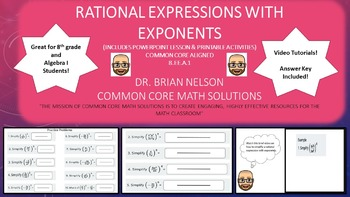# Rational Expressions with Exponents - PowerPoint Lesson and Printables!Subject
Resource Type
File Type

Presentation (Powerpoint) File

(45 MB|9 pages)
Product Rating
Standards
• Product Description
• StandardsNEW
This purchase includes a PowerPoint lesson on rational expressions with exponents. The PowerPoint is designed for whole classroom instruction while the pages may be individually printed as worksheets. The PowerPoint includes an answer key and video solutions.

Objectives

Accurately distribute an exponent to all components of an expression.

Essential Questions

How do we apply the properties of exponents when distributing to simplify an equation?

Key Concepts

When a rational expression is raised to an exponent, that exponent is applied to both the numerator and the denominator.

Standards

CCSS.Math.Content.8.EE.A.1 Know and apply the properties of integer exponents to generate equivalent numerical expressions. For example, 3² × 3⁻⁵ = 3⁻³ = (1/3)³ = 1/27.
Know and apply the properties of integer exponents to generate equivalent numerical expressions. For example, 3² × (3⁻⁵) = (3⁻³) = 1/3³ = 1/27.
Total Pages
9 pages
Included
Teaching Duration
50 minutes
Report this Resource to TpT
Reported resources will be reviewed by our team. Report this resource to let us know if this resource violates TpT’s content guidelines.